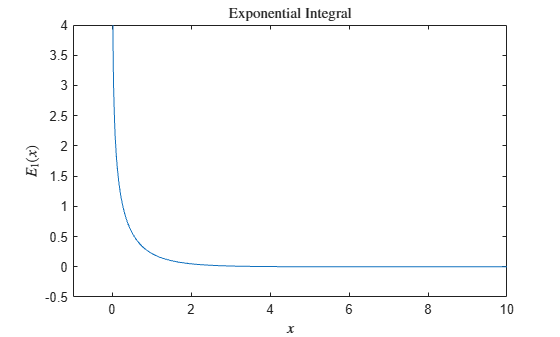# expint

Exponential integral function

## Syntax

``Y = expint(X)``

## Description

example

````Y = expint(X)` evaluates the exponential integral for each element of `X`.```

## Examples

collapse all

Find the exponential integral for `X` `=` `1+2i`.

`Y = expint(1+2i)`
```Y = -0.1268 - 0.0351i ```

Plot the exponential integral for `X` in the interval of `[0,10]`.

```X = 0:0.01:10; Y = expint(X); plot(X,Y) axis([-1 10 -0.5 4]) xlabel('\$x\$','interpreter','latex') ylabel('\$E_1(x)\$','interpreter','latex') title('Exponential Integral','interpreter','latex')```## Input Arguments

collapse all

Input array, specified as a scalar, vector, matrix, or multidimensional array.

Data Types: `single` | `double`
Complex Number Support: Yes

collapse all

### Exponential Integral

The exponential integral of x is defined as

By analytic continuation, `expint` is a scalar-valued function in the complex plane cut along the negative real axis.

There is a different function that is sometimes called the exponential integral: the Cauchy principal value integral

which, for positive real x, is related to `expint` as

`$\underset{\delta \to 0+}{\mathrm{lim}}{E}_{1}\left(-x+i0\right)=-\text{Ei}\left(x\right)-i\pi .$`

 Abramowitz, M. and I. A. Stegun. Handbook of Mathematical Functions. Chapter 5, New York: Dover Publications, 1965.